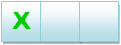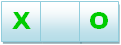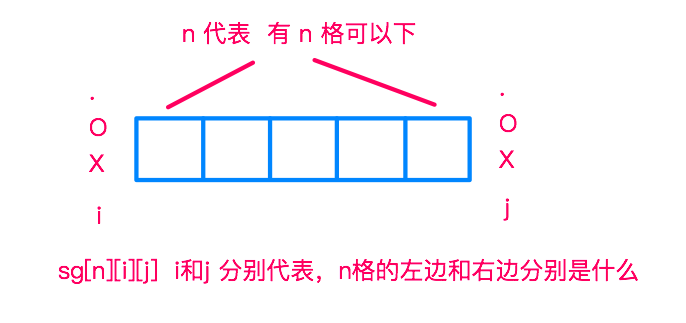# ACM | LightOJ 1401 No More Tic-tac-toe 博弈论SG打表

### No More Tic-tac-toe

##### Problem Description

Alice was bored with the game tic-tac-toe. Usually she used to play against computer. She was so bored because, she always managed to make a tie against computer as she already became an expert in this game. She was also bored about the matching things, that means she does not like to see two adjacent 'X's or two adjacent 'O's. So Bob created a new computer game for her.
Here is the description of this new 2-player game:

1. A 1 x N grid will be given.
2. In each turn, a player can mark an unmarked cell by an 'X' or by an 'O' (it's not Zero it is big 'O').
3. No two adjacent 'X's are allowed in this game.
4. No two adjacent 'O's are allowed in this game.
5. The player who marks the last cell wins the game.
Let's have an example for N = 3. Suppose first player mark the leftmost cell with an 'X'. Then the grid will look like this:From this state, the winning move of the second player is to mark the rightmost cell with an 'O'.Now first player has no move, because in the only empty cell she cannot mark 'X' and cannot mark 'O' because the left of this cell is already marked 'X' and the right of this cell is marked 'O'. The second player will win.

But this is not an optimal example for first player. If in first move, the first player marks the middle cell with any one of 'X' or 'O', she will win.

Now about Bob's computer game, Alice always makes the first move. Alice usually used to start playing with Bob. But most often after some move, Bob gave his responsibility to the computer. Computer always plays optimally. You are given the state of the grid after Bob's departure and your task is to determine whether it is possible to win against computer from this state for Alice.

##### Input

Input starts with an integer T (≤ 10000), denoting the number of test cases.

Each case starts with a line containing a string which represents the state of the game after Bob's departure. Here the length of the string is N (1 ≤ N ≤ 100). In this string besides 'X' and 'O', another character '.' is used, which represents an unmarked cell.

##### Output

For each case, print the case number and 'Yes' if it is possible to win against computer, otherwise print 'No'.

5
...
X..
....O
..X..
O

Case 1: Yes
Case 2: No
Case 3: No
Case 4: Yes
Case 5: Yes

##### SG打表##### 打表+代码:
#include <cstdio>
#include <cstring>
using namespace std;
const int maxn = 110;

int sg[maxn]; // 0->.  1->O  2->X
int vis[maxn];

void init() {
for (int i = 1; i < maxn; ++i) {
for (int j = 0; j < 3; ++j) {
for (int k = 0; k < 3; ++k) {
memset(vis, 0, sizeof(vis));
for (int pos = 1; pos <= i; ++pos) {
if (!((pos == 1 && j == 1)
|| (i == pos && 1 == k))) {
vis[sg[pos - 1][j] ^ sg[i - pos][k]] = 1;
//printf("%d %d\n", sg[pos - 1][j], sg[i - pos][k]);
}
if (!((pos == 1 && j == 2)
|| (i == pos && k == 2))) {
vis[sg[pos - 1][j] ^ sg[i - pos][k]] = 1;
//printf("%d %d\n", sg[pos - 1][j], sg[i - pos][k]);
}
}
for (int t = 0; ; ++t) {
if (vis[t] == 0) {
sg[i][j][k] = t;
//if (j > 0 && k > 0) printf("%d %d %d %d\n", i, j, k, t);
break;
}
}
}
}
}
}

int main() {
init();
int t, ca = 1;
char s[maxn];
scanf("%d", &t);
while (t--) {
scanf("%s", s);
int num = 0, last = 0, lc = 0, ans = 0;
for (int i = 0; s[i]; ++i) {
if (s[i] != '.') {
++num;
int nowc = s[i] == 'O' ? 1 : 2;
ans ^= sg[i - last][lc][nowc];
lc = nowc, last = i + 1;
}
}
ans ^= sg[strlen(s) - last][lc];
if (num % 2 == 0) {
if (ans == 0) printf("Case %d: No\n", ca++);
else printf("Case %d: Yes\n", ca++);
} else {
if (ans != 0) printf("Case %d: No\n", ca++);
else printf("Case %d: Yes\n", ca++);
}
}
return 0;
}